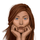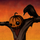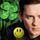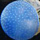## General Question# Why is the Pythagorean theorem true?

Asked by Soubresaut (13707) August 14th, 2020

I know several ways to show that it’s true. I’m not so much interested in ways of proving the truth of the theorem as I am in trying to understand in a physical way why it’s true.

Why are the sides of a right triangle related to each other that way?

Observing members: 0Composing members: 0“The principles of logic and mathematics are true simply because we never allow them to be anything else. And the reason for this is that we cannot abandon them without contradicting ourselves.”
—A.J. Ayer

SavoirFaire (27671)“Great Answer” (5) Flag as…Here is a neat physical proof called the Pythagorean Theorem Rearranment Proof .
Elegant
You can also find it on youtube.

Thanks for the Q. I did not think to prove it this way. I had to resort to math which would not have answered the spirit of your question.

LuckyGuy (40078)“Great Answer” (6) Flag as…In mathematical philosophy, there is discussion of when a proof can be considered explanatory. Link It seems your question is related to this. One thing that I find annoying about all the proofs of the Pythagorean Theorem that I have seen is that they all involve areas. Why should a proof regarding lengths require information about areas?

Having said that, there is a very simple proof of the Pythagoean Theorem due to Einstein when he was twelve years old.

Preliminary:
It can be shown that for any triangle and all triangles similar to it, taking corresponding sides for all these triangles, the areas are kx^2 where k is a constant and x is the length of the corresponding side.

Einstein’s proof:
Take any right triangle and split it into two triangles by drawing the perpendicular segment from the right angle to the hypotenuse. The two smaller triangles are similar to the larger triangle and have hypotenuses of a and b. There is some k such that the area of the larger triangle is kc2, which means that the areas of the two smaller triangles are ka2 and kb2. Since the area of the larger triangle is the sum of the areas of the two smaller triangles, we have kc2 = ka2 + kb2 and then c2 = a2 + b^2.

LostInParadise (29006)“Great Answer” (2) Flag as…There are a number of constraints that the formula for the hypotenuse must satisfy. It is easy to show that the Pythagorean equation satisfies all of them.

Euclidean geometry requires that the side opposite the largest angle of a triangle must be the largest side. Since the 90 degree angle is always the largest angle, we must have sqrt(a**2+b**2) > a and > b

The triangle inequality requires that a+b > sqrt(a**2 + b**2)

If we have a triangle with legs a and b and hypotenuse c, and another triangle with legs ka and kb, then the two triangles are similar, meaning that the second triangle must have a hypotenuse of kc. c**2 = a**2 + b**2 must imply that (kc)***********2 = (ka)********2 + (kb)***2

Since there is no way of distinguishing which leg is a and which is b, the formula must be symmetric in a and b. c**2 = 2a***2 + b**2 would violate this constraint.

The units on the left side of the equation must match those on the right. The left side has units of length squared and so does the right. c**3 = a**2+b**2 would violate this constraint.

LostInParadise (29006)“Great Answer” (2) Flag as…Sorry for all those *‘s. It is hard to indicate exponentiation.

LostInParadise (29006)“Great Answer” (1) Flag as…@LostInParadise “It is hard to indicate exponentiation.”

The caret or circumflex sign is how exponentiation is indicated.

eg. x^2 is x squared.

“Why are the sides of a right triangle related to each other that way?”

Because if you constrain one angle to be equal to 90 degrees then the other two angles will add up to 90 degrees. A concomitant is that the sum of the squares on those sides equal the square on the hypotenuse.

doyendroll (1771)“Great Answer” (1) Flag as…Thank you all again! I’m still chewing on this one…

@LostInParadise—thank you for mentioning and linking to discussion about the difference between “proof” and “explanation.” I think that is the distinction I’m trying to get at here—not so much have it proved true, but an explanation of it.

I think, too, that part of my challenge in understanding an explanation is probably that I’m not well versed in math as a discipline. So you and @LuckyGuy may well have provided explanations that I’m just not understanding. I’m playing around with them to see what I can make sense of in a way that means more to me at my level of understanding.

Soubresaut (13707)“Great Answer” (0) Flag as…Let me know if you have any questions about the proof I showed. Like I said, I don’t think that proofs using areas are good explanations of the Pythagorean Theorem, but if you are going to do such a proof, Einstein’s method of proof is short and sweet.

LostInParadise (29006)“Great Answer” (1) Flag as…@LostInParadise—My question about the Einstein one would be this: Is there not a way to split any triangle into two similar triangles, and so be able to describe any triangle in that way? I’ve been very roughly sketching them and it seems like I can (but I’m just eyeballing sketches, so maybe not). The line wouldn’t be perpendicular (it would be an angle particular to the original triangle), and there isn’t technically a hypotenuse (just the longest side), but if the three triangles are similar, then isn’t there still some k that relates a2, b2, and c^2 each to the area of their respective triangle? (I’m presuming this isn’t actually correct, so why not? How is it different for a right triangle compared to others?)

I have a related question with yours, @LuckyGuy—I’ll address it to whoever wants to answer: I was trying to play around with using parallelograms instead of rectangles. Basically, I seem to get negative space in the first version with 2 rhombuses, one with side lengths “a” and one with side lengths “b.” When I split the parallelograms and rearrange, I get a parallelogram with side lengths “c” and “d” (because the parallelograms are split along different diagonals). It seems I still get a relationship between the squares of the sides of the triangles. That is, that: a*(height of rhombus a) + b*(height of rhombus b) = c*(height of parallelogram with c as base) = d*(height of parallelogram with d as base).... but in the case of a right triangle, the height is the same as the side length and so things simplify down. Is that true? And if so, is there a more complex/complete version of the idea behind the Pythagorean equation that describes the relationship between the sides of any triangle, provided you know the relevant angle? (Or again, is this not actually correct? And why not?... if it is true, it does seem less immediately useful—would have to know more about the triangle—but would make the Pythagorean equation seem less like it’s out of nowhere).

Soubresaut (13707)“Great Answer” (0) Flag as…In answer to your question, the smaller triangles are not just similar to each other, but to the larger triangle as well. I don’t think this can be done unless you drop a perpendicular from a right angle. Suppose you drop a line from some angle to the opposite side. The two angles at the opposite side have to add to 180. Suppose the two angles are 110 and 70. Then the two small triangles, in order to be similar, would need to both have angles of 70 and 110, since similar triangles have identical angles. That is impossible, since the three angles of a triangle add to 180. With 90 degrees, you just need each small triangle to have a 90 degree angle.

LostInParadise (29006)“Great Answer” (1) Flag as…or